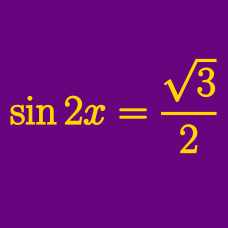Geometry

Trigonometric Equations: Level 2 Challenges

Find all values of $\theta$ such that

$\cos^2 \theta = 1.$

In the options, $n$ is an integer.

Given that

$\cos(A)-4\sin(A)=1,$

what are the possible values of

$\sin(A) + 4\cos(A)?$

$\sin\theta+\cos\theta=\sqrt{2}\sin(90^{\circ}-\theta), \quad \cot\theta = \, ?$

Give your answer to the above problem to 3 decimal places.

Find the value of $x$ between 0 and 180 such that

$\tan({ 120 }^{ \circ }-x^{ \circ })=\frac { \sin{ 120 }^{ \circ }-\sin x^{ \circ } }{ \cos{ 120 }^{ \circ }-\cos x^{ \circ }}.$

If $\quad \quad \dfrac{\cos^4\alpha}{\cos^2\beta}+\dfrac{\sin^4\alpha}{\sin^2\beta}=1,$ find the value of $\dfrac{\sin^4\beta}{\sin^2\alpha}+\dfrac{\cos^4\beta}{\cos^2\alpha}.$

×